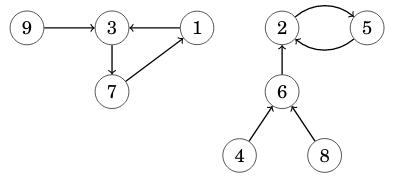CSES - Functional Graph Distribution
• Time limit: 1.00 s
• Memory limit: 512 MB

A functional graph is a directed graph where each node has outdegree 1. For example, here is a functional graph that has 9 nodes and 2 components:Given n, your task is to calculate for each k=1 \dots n the number of functional graphs that have n nodes and k components.

# Input

The only input line has an integer n: the number of nodes.

# Output

Print n lines: for each k=1 \dots n the number of graphs modulo 10^9+7.

# Constraints

• 1 \le n \le 5000

# Example

Input:

3


Output:

17
9
1